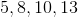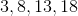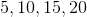# High School Math : Understanding Arithmetic Series

## Example Questions

### Example Question #1 : Arithmetic Series

List the first 4 terms of an arithmetic sequence with a first term of 3, and a common difference of 5.Explanation:

An arithmetic sequence is one in which the common difference is added to one term to get the next term. Thus, if the first term is 3, we add 5 to get the second term, and continue in this manner.

Thus, the first four terms are:### All High School Math Resources